# What is the maximum air compression

## Pneumatics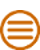The following formulas and tables are non-binding and are intended to facilitate the rough design of a pneumatic system.

up

up

### Standard volume - Vn - Nm3

The standard volume is set to a value of pressure = 101325 Pa = 1.01325 barSectionbased
According to DIN 1343, the temperature refers to = 273.15 K = 0 ° C
According to DIN 1343, the standard volume is approx. 8% smaller than at 20 ° C.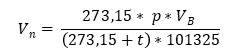V.n = Standard volume (m3)
V.B. = Operating volume (m3)
p = absolute pressure (Pa)
t = temperature (° C)
V.n = Standard volume (m3)
V.B. = Operating volume (m3)
p = absolute pressure (Pa)
t = temperature (° C)
up

### Operating volume - VB. - m3

The operating volume is based on the actual condition.
The temperature, air pressure and humidity must be taken into account.
The pressure must always be specified for the operating volume.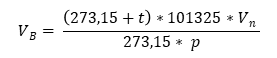V.n = Standard volume (m3)
V.B. = Operating volume (m3)
p = absolute pressure (Pa)
t = temperature (° C)
V.n = Standard volume (m3)
V.B. = Operating volume (m3)
p = absolute pressure (Pa)
t = temperature (° C)
up

### Volume flow - V - l / min, m3/ min, m3/H

A volume flow measured on the pressure side is calculated back to the suction state in compressed air technology in order to be able to compare the compressors with one another.
When converting to the intake condition, the relaxed intake pressure, intake temperature and air humidity must be taken into account.

### Standard volume flow - Qn - l / min, m3/ min, m3/H

The standard volume flow does not refer to the intake condition, but to a physical standard condition with the following values.
Temperature = 273.15 K
Pressure = 101325 Pa
Air density = 1.294 kg / m3 (dry air)
rel. Humidity = 0%

### Operating volume flow - QB. - l / min, m3/ min, m3/H

The operating volume flow indicates the effective volume flow of the compressed air.
In order to be able to compare the operating volume flow, the pressure must always be specified.

up

### Atmospheric pressure - pamb - Pa

Atmospheric pressure is the air pressure on site. It depends on the density and the height.
The air pressure decreases with increasing altitude. The following pressure applies at sea level:
pamb = 101325 Pa = 1.01325 bar = 760 mm / Hg (Torr)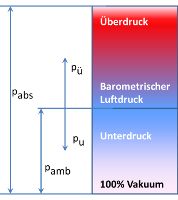### Overpressure - p - Pa

In compressed air technology, the pressure is usually given as overpressure, without the index "ü".

### Absolute pressure - pSection - Pa

The absolute pressure is the sum of atmospheric pressure pamb and the overpressure pü
The pressure is given in Pascal (Pa) according to the SI system
1 Pascal = 1 Newton / 1 m2 = 1 N / 1 m2

up

### Pressure areas

In compressed air technology, the pressure ranges are divided as follows:

### Low pressure range up to 10 bar

Application in industry and craft.
Used compressors:
- one- and two-stage piston compressors
- one and two-stage screw compressors
- rotary compressor

### Medium pressure range up to 15 bar

Application area for truck and heavy-duty vehicle tires as well as special machines.
Used compressors:
- two-stage piston compressors
- single-stage screw compressors, oil-injection cooled

### High pressure range up to 40 bar

Area of ​​application for starting large diesel engines or for pressure testing of pipelines.
Used compressors:
- two- and three-stage piston compressors
- multi-stage screw compressors

### High pressure range up to 400 bar

Area of ​​application for storing breathing air in diving bottles, in power plants, rolling mills and smelting works for leak tests.
Used compressors:
- three- and four-stage piston compressors

up

### Water in the compressed air

There is always a certain amount of water vapor in the atmospheric air. The content is referred to as air humidity (moisture). At any temperature, a given volume of air can only contain a maximum amount of water vapor.

up

### Saturation vapor pressure

The maximum possible water vapor pressure at a certain temperature is called the saturation vapor pressure. The following Magnus formula is generally used to calculate the saturation vapor pressure above water.

Validity range: -45 ° C ≤ t ≤ 60 ° C
Magnus formula over water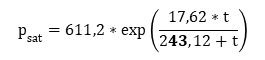psat = Saturation vapor pressure (Pa)
t = air temperature (° C)
psat = Saturation vapor pressure (Pa)
t = air temperature (° C)
up

### Maximum humidity - fMax - g / m3

Below the maximum humidity fMax (Saturation amount) means the maximum amount of water vapor that 1 m³ of air can contain at a certain temperature.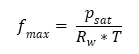fMax = max. humidity (g / m³)
psat = Saturation vapor pressure (Pa)
t = air temperature (° C)
R.w = Gas constant water vapor = 461.51 (J / (kg * K))
fMax = max. humidity (g / m³)
psat = Saturation vapor pressure (Pa)
t = air temperature (° C)
R.w = Gas constant water vapor = 461.51 (J / (kg * K))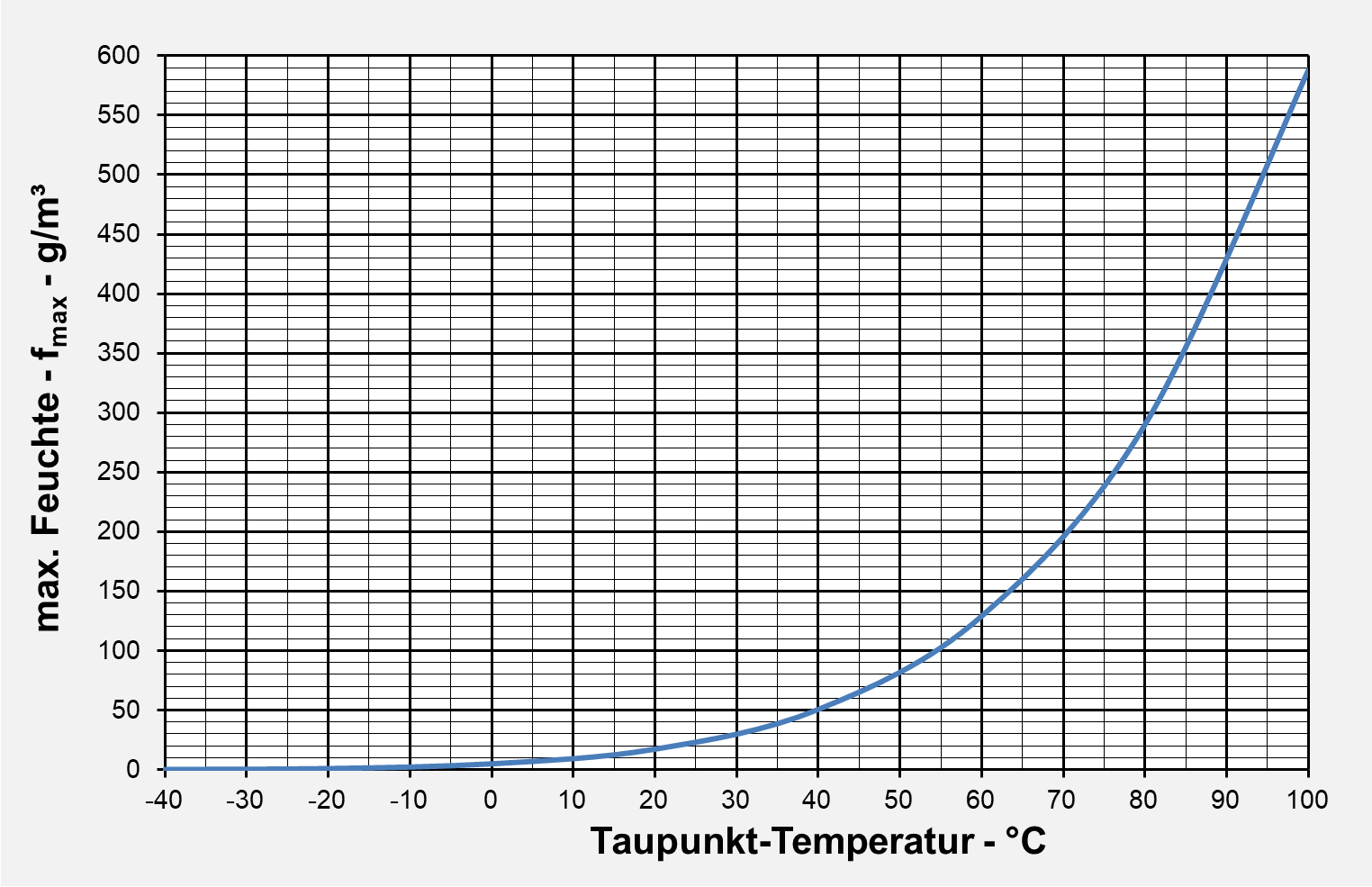up

### Relative humidity - φ -%

The relative humidity φ is understood to be the ratio of the absolute to the maximum humidity.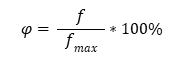φ = humidity (%)
f = absolute humidity (g / m3)
fMax = maximum humidity (g / m3)
φ = humidity (%)
f = absolute humidity (g / m3)
fMax = maximum humidity (g / m3)

Since the maximum humidity fMax is temperature-dependent, the relative humidity changes with the temperature, even if the absolute humidity remains constant. When it cools down to the dew point, the relative humidity increases to 100%.

up

### Absolute humidity - f - g / m3

The absolute humidity f is the amount of water vapor actually contained in 1 m³ of air.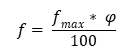f = absolute humidity (g / m3)
fMax = maximum humidity (g / m3)
φ = humidity (%)
f = absolute humidity (g / m3)
fMax = maximum humidity (g / m3)
φ = humidity (%)
up

### Water content in uncompressed airmW. = Water content (g / h)
V = air volume flow (m3/H)
fMax = maximum humidity (g / m3)
φ = relative humidity (%)
mW. = Water content (g / h)
V = air volume flow (m3/H)
fMax = maximum humidity (g / m3)
φ = relative humidity (%)
up

### Amount of condensate during compression

Air always contains water in the form of steam. Since air, unlike water, is compressible, the water precipitates out in the form of condensate when it is compressed. The maximum humidity of the air is temperature and volume dependent. It is not quantity-dependent.

Loss of condensate volume when air is compressed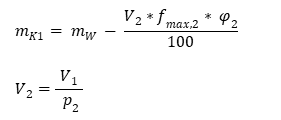mK1 = Precipitated condensate (g / h)
mw = Water content of uncompressed air (g / h)
V.1 = Volume flow initial state (m3/H)
V.2 = Volume flow in compressed state (m3/H)
fmax, 2 = maximum moisture in the compressed state (g / m3)
φ2 = relative humidity when compressed - 100% (%)
mK1 = Precipitated condensate (g / h)
mw = Water content of uncompressed air (g / h)
V.1 = Volume flow initial state (m3/H)
V.2 = Volume flow in compressed state (m3/H)
fmax, 2 = maximum moisture in the compressed state (g / m3)
φ2 = relative humidity when compressed - 100% (%)

Since only the water that cannot be stored falls out of the compressed air, the relative humidity φ of the compressed air rises to 100%.

up

### Amount of condensate after the dryer

Compressed air in the refrigeration compressed air dryer is cooled down, causing the pressure dew point to drop and the condensate to precipitate.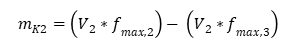mK2 = Precipitated condensate (g / h)
V.2 = Volume flow in compressed state (m3/H)
fmax, 2 = maximum moisture after compression (g / m3)
fmax, 3 = maximum moisture after the dryer (g / m3)
mK2 = Precipitated condensate (g / h)
V.2 = Volume flow in compressed state (m3/H)
fmax, 2 = maximum moisture after compression (g / m3)
fmax, 3 = maximum moisture after the dryer (g / m3)
up

### Atmospheric dew point - ° C

Atmospheric dew point is the temperature at which atmospheric air (1 barSection) can be cooled without water precipitating.

Validity range: -45 ° C ≤ t ≤ 60 ° C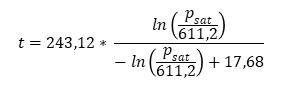t = atmospheric dew point temperature (° C)
psat = Saturation vapor pressure (Pa)
t = atmospheric dew point temperature (° C)
psat = Saturation vapor pressure (Pa)
up

### Pressure dew point temperature - ° C

The pressure dew point is the temperature to which compressed air can be cooled without condensate forming. The pressure dew point depends on the final compression pressure. When the pressure drops, the pressure dew point also drops.

Validity range: -45 ° C ≤ t ≤ 60 ° C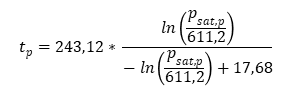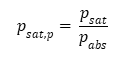tp = Pressure dew point temperature (° C)
psat, p = Saturation vapor pressure under pressure p (Pa)
psat = Saturation vapor pressure at 1 barSection(Pa)
pSection = Absolute pressure (barSection)
tp = Pressure dew point temperature (° C)
psat, p = Saturation vapor pressure under pressure p (Pa)
psat = Saturation vapor pressure at 1 barSection(Pa)
pSection = Absolute pressure (barSection)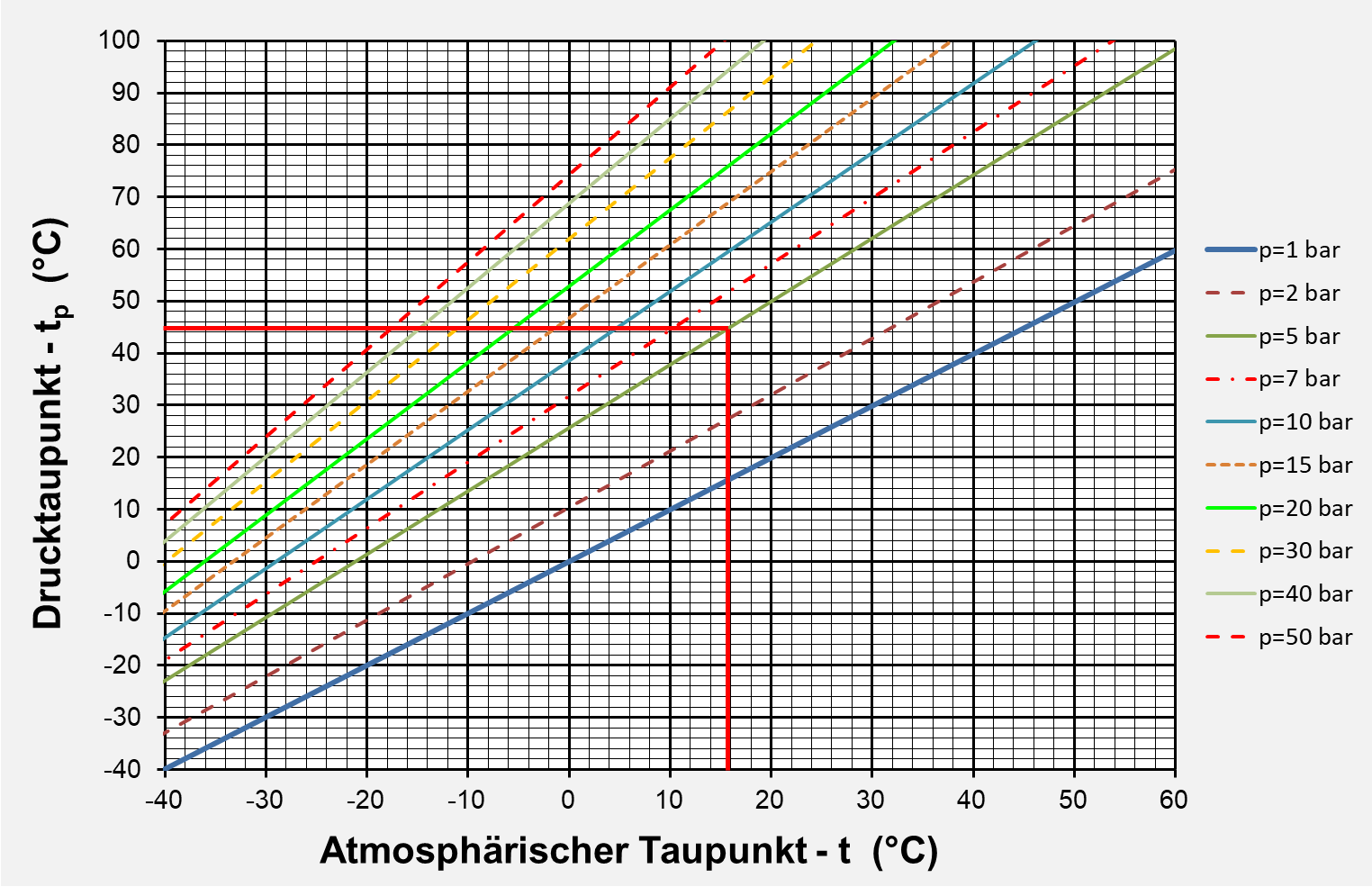Example: Atmosph. Dew point t = 16 ° C - pressure pSection = 5 bar - pressure dew point tp = 45 ° C

up

### Compressed air quality according to ISO 8573.1

max.
size
(μm)
max.
density
(mg / m3)
Water content
max.

Class: 1 z. B. Photo industry / 2 z. B. aviation / 3 z. B. Packaging Industry /
4 z. B. General Industry / 5 z. B. Mining

up

### Compressed air consumption by nozzles

Reference values ​​for the compressed air consumption of cylindrical nozzles (blow guns) depending on the working pressure and nozzle diameter.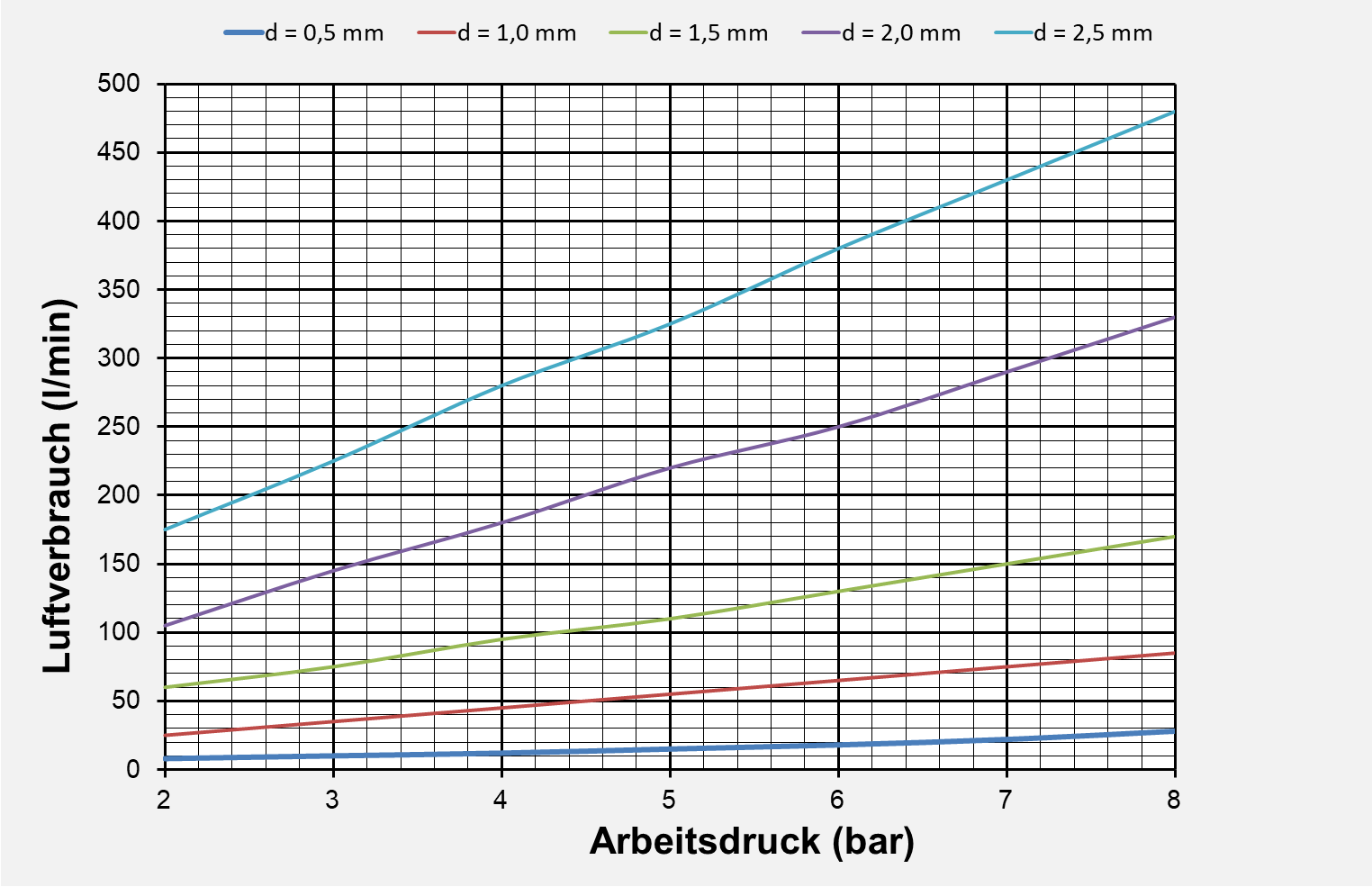up

### Compressed air consumption for paint spray guns

Reference values ​​for the compressed air consumption of paint spray guns depending on the working pressure and nozzle diameter for flat and wide jet nozzles.up

### Compressed air consumption for blasting nozzles

Reference values ​​for the compressed air consumption of blasting nozzles depending on the working pressure and nozzle diameter.up

### Compressed air consumption of cylinders

Single-acting cylinders only require compressed air for the working stroke. The reset takes place by external or spring force.
Double-acting cylinders require compressed air for the working stroke and the return of the cylinder.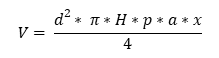V = air consumption (l / min)
d = piston diameter (dm)
H = piston stroke (dm)
pSection = Operating pressure (barSection
a = work cycles per minute (1 / min)
x = 1 single-acting cylinder
x = 2 double-acting cylinders
V = air consumption (l / min)
d = piston diameter (dm)
H = piston stroke (dm)
pSection = Operating pressure (barSection
a = work cycles per minute (1 / min)
x = 1 single-acting cylinder
x = 2 double-acting cylinders
up

### Compressed air consumption of tools

Reference values ​​for the compressed air consumption of compressed air tools.

4 mm
4 ... 10 mm
10 ... 32 mm

Plastic and textile scissors

lightweight demolition and breaker hammer

Rammers - concrete and earth

up

### Medium duty cycle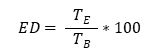ED = mean Duty cycle (%)
TE. = Switch-on time (min)
TB. = Reference time (min)
ED = mean Duty cycle (%)
TE. = Switch-on time (min)
TB. = Reference time (min)
up

### Simultaneity factor

The simultaneity factor takes into account that not all consumers are in use at the same time. The factor is an empirical value that is taken into account in the case of non-automatic consumers.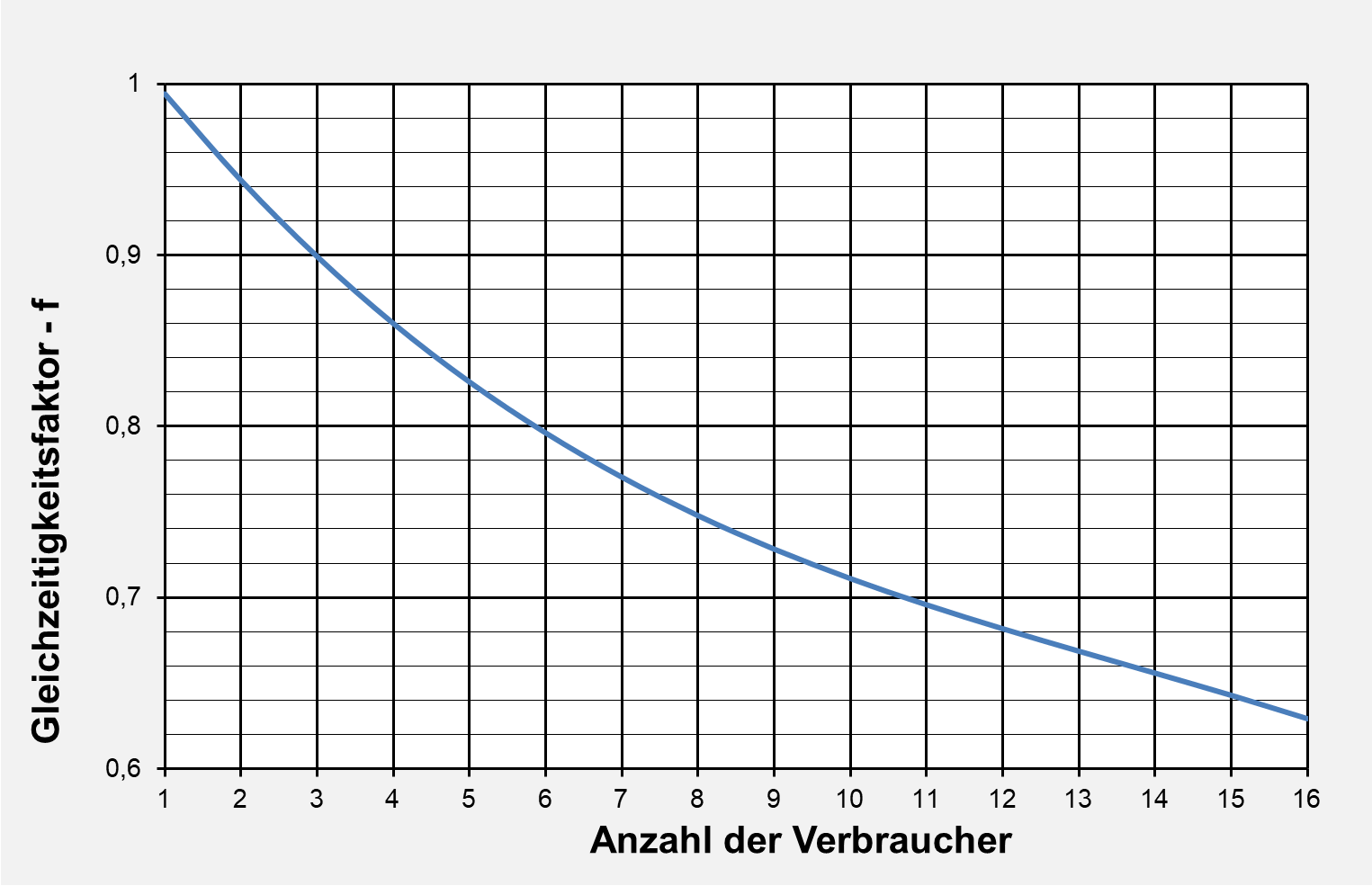up

### Total compressed air requirement

When determining the compressed air requirement, the consumers are divided into two groups.
- Automatic consumers with continuous work processes.
- General consumers with only a temporary switch-on time.
The compressed air requirement is determined as follows: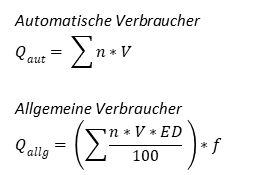Qaut = Total air requirement for automatic consumers (l / min)
Qgeneral = Total air requirement for general consumers (l / min)
n = number of identical consumers (-)
V = individual consumption (l / min)
ED = mean Duty cycle (%)
f = simultaneity factor
Qaut = Total air requirement for automatic consumers (l / min)
Qgeneral = Total air requirement for general consumers (l / min)
n = number of identical consumers (-)
V = individual consumption (l / min)
ED = mean Duty cycle (%)
f = simultaneity factor
up

### Losses, reserves, surcharges

The following surcharges are taken into account for the realistic total air consumption

Losses v -%

Approx. 5% to 25% of the total delivery quantity must be taken into account for losses due to leakage and friction.

Reserves r -%

For later additional consumers, at least 10% and possibly up to 100% of the total delivery quantity will be taken into account.

Misjudgments x -%

On the basis of many assumptions, approx. 5% to 15% of the total delivery quantity must be taken into account.

up

### quantity delivered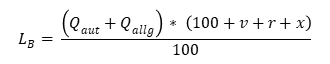L.B. = Delivery quantity (l / min)
Qaut = Total air requirement for automatic consumers (l / min)
Qgeneral = Total air requirement for general consumers (l / min)
v = losses (%)
r = reserves (%)
x = misjudgments (%)
L.B. = Delivery quantity (l / min)
Qaut = Total air requirement for automatic consumers (l / min)
Qgeneral = Total air requirement for general consumers (l / min)
v = losses (%)
r = reserves (%)
x = misjudgments (%)
up

### Compressor types

Plunger Compressor
10 (1-stage)
35 (2-stage)

Liquid ring compressor

Axial and centrifugal compressors

up

The following factors are decisive for determining the size of the compressor station:

### Working pressure - pA. - bar

The working pressure is the pressure that consumers need. The minimum pressure in the compressed air network should always be above the working pressure.

### Cut-out pressure - pMax - bar

The switch-off pressure is the maximum pressure in the compressed air network. When the switch-off pressure is reached, the compressor is switched off.
When determining the working pressure pMax to consider: At normal compressed air networks the sum of the pressure losses Δp should not exceed 0.1 bar.
At Large compressed air networks a pressure loss Δp of up to 0.5 bar is possible.
Compressed air treatment by dryer:
- Membrane compressed air dryer with filter ≤ 0.6 bar
- Cold compressed air dryer ≤ 0.2 bar
- Adsorption compressed air dryer with filter ≤ 0.8 bar
Compressed air treatment through filters ≤ 0.6 bar
Switching differential of the compressor
- Screw compressors 0.5 ... 1 bar
- reciprocating compressors pMax - 20%

### Switching differential - Δp - bar

The switching difference is the pressure difference between pMax and pmin.

up

### Compressed air tank volume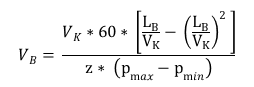V.B. = Volume of the compressed air tank (m3)
V.K = Delivery volume of the compressor (m3/ min)
L.B. = Required delivery quantity (m3)
z = permissible motor switching cycles (1 / h)
pMax = Switch-off pressure of the compressor (bar)
pmin = Switch-on pressure of the compressor (bar)
V.B. = Volume of the compressed air tank (m3)
V.K = Delivery volume of the compressor (m3/ min)
L.B. = Required delivery quantity (m3/ min)
z = permissible motor switching cycles (1 / h)
pMax = Switch-off pressure of the compressor (bar)
pmin = Switch-on pressure of the compressor (bar)
up

### Compressor downtime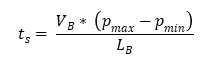tS. = Compressor downtime (min)
V.B. = Volume of the compressed air tank (l)
L.B. = Required delivery quantity (l / min)
pMax = Switch-off pressure of the compressor (bar)
pmin = Switch-on pressure of the compressor (bar)
tS. = Compressor downtime (min)
V.B. = Volume of the compressed air tank (l)
L.B. = Required delivery quantity (l / min)
pMax = Switch-off pressure of the compressor (bar)
pmin = Switch-on pressure of the compressor (bar)
up

### Compressor running time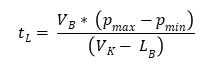tL. = Compressor running time (min)
V.B. = Volume of the compressed air tank (l)
L.B. = Required delivery quantity (l / min)
V.K = Delivery volume of the compressor (l / min)
pMax = Switch-off pressure of the compressor (bar)
pmin = Switch-on pressure of the compressor (bar)
tL. = Compressor running time (min)
V.B. = Volume of the compressed air tank (l)
L.B. = Required delivery quantity (l / min)
V.K = Delivery volume of the compressor (l / min)
pMax = Switch-off pressure of the compressor (bar)
pmin = Switch-on pressure of the compressor (bar)
up

### Compressor switching cycles

The switching cycles depend on the size of the drive motor.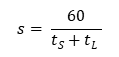s = compressor switching cycles (1 / h)
tL. = Running time of the compressor in one hour (min)
tS. = Downtime of the compressor in one hour (min)
s = compressor switching cycles (1 / h)
tL. = Running time of the compressor in one hour (min)
tS. = Downtime of the compressor in one hour (min)
Guide values ​​for the permissible switching cycles of a drive motor.
 Motor power (kW) perm motor switching cycles s (1 / h) 4...7,5 30 11...22 25 30..55 20 65...90 15 110...160 10 200...250 5
up

### Maximum pressure drop

The following values ​​should not be exceeded as the maximum pressure loss at a maximum pressure of 8 bar and more:
- entire pipeline network Δp ≤ 0.1 bar
- Main line Δp ≤ 0.04 bar
- Distribution line Δp ≤ 0.04 bar
- Connection line Δp ≤ 0.03 bar
If the maximum pressure is lower, the following pressure loss can be assumed:
- entire pipeline network Δp ≤ 1.5% * pMax bar

up

### Equivalent pipe length for fittings

The pipe length is decisive for determining the pipe diameter for a given pressure loss. The built-in valves, elbows and fittings are added to the total pipe length by adding an equivalent pipe length.
The equivalent pipe lengths for fittings can be found in tables in the general literature or in the manufacturer's catalogs for compressed air systems. These values ​​are only suitable for an approximate pipe dimensioning, as there is usually no information on the underlying flow velocity. A more precise calculation of the pressure loss of fittings see below.
The following formula can be used as the basis for the equivalent pipe length.
The calculation is made using the zeta value of the component or the pipe friction coefficient. The pipe friction coefficient is obtained from the Moody diagram, in which the pipe friction coefficient can be read off as a function of the Reynolds number and the ratio of the pipe inner diameter to the pipe roughness. The zeta values ​​are listed in the manufacturer's catalog or can be found in the following zeta value overview.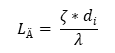L.Ä = equivalent pipe length (m)
ζ = zeta value (-)
di = Pipe inside diameter (m)
λ = pipe friction coefficient (-)
L.Ä = equivalent pipe length (m)
ζ = zeta value (-)
di = Pipe inside diameter (m)
λ = pipe friction coefficient (-)
up

### Rough determination of the pipe inside diameter

The pipe diameter can be determined with the approximation formula. The delivery quantity of the compressor is to be specified in the intake state upstream of the compressor. This calculation is based on a pipe roughness of 0.1 mm.di = Pipe inside diameter (m)
V.K = Delivery volume of the compressor (m³ / s)
L = total pipe length with equivalent portion LÄ (m)
Δp = permissible pressure drop (bar)
pMax = Switch-off pressure of the compressor (bar)
di = Pipe inside diameter (m)
V.K = Delivery volume of the compressor (m³ / s)
L = total pipe length with equivalent portion LÄ (m)
Δp = permissible pressure drop (bar)
pMax = Switch-off pressure of the compressor (bar)
up

### Calculation of pressure loss

The total pressure loss can be calculated using the following approximation formula.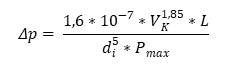Δp = pressure loss (bar)
V.K = Delivery volume of the compressor (m³ / s)
L = total pipe length with equivalent portion LÄ (m)
di = Pipe inside diameter (m)
pMax = Switch-off pressure of the compressor (bar)
Δp = pressure loss (bar)
V.K = Delivery volume of the compressor (m³ / s)
L = total pipe length with equivalent portion LÄ (m)
di = Pipe inside diameter (m)
pMax = Switch-off pressure of the compressor (bar)
up

A more precise calculation of the pressure loss in a straight pipe is possible with the following calculation program.
The program takes into account the expansion flow, as well as the density and viscosity of the air, is taken into account depending on the pressure and temperature. Enter the compressed air flow for the volume flow.

The pressure loss of the fittings must then be calculated using the following formula.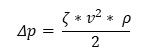Δp = valve pressure loss (Pa)
ζ = zeta value of the valve (-)
v = flow velocity (m / s)
ρ = density of air (kg / m³)
Δp = valve pressure loss (Pa)
ζ = zeta value of the valve (-)
v = flow velocity (m / s)
ρ = density of air (kg / m³)
up

up

up

### traction

Pulling force of double-acting cylinders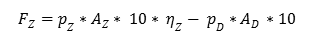F. Z = Tensile force (N)
p Z = Pressure pull side (bar)
p Z results from line and valve resistance of the outflowing air
A. Z = Piston area traction side (cm²)
A. D. = Piston area pressure side (cm²)
η K = Piston efficiency (-)
F. Z = Tensile force (N)
p Z = Oil pressure pull side (bar)
p Z results from line and valve resistance of the outflowing air
A. Z = Piston area traction side (cm²)
A. D. = Piston area pressure side (cm²)
η K = Piston efficiency (-)
up

### Efficiency of the piston

The efficiency η of the piston due to friction and leakage is in the range of approx. 0.85-0.95.
The exact values ​​can be found in the manufacturer's information.
It must be taken into account that new pistons have a higher frictional force due to the new seal, and thus have a poorer efficiency.

up

### Buckling of the piston rod

The buckling of the piston rod must be calculated for the elastic area, i.e. H. for the Euler case. Whether the Euler case applies can be checked using the formula given.
A value of 3 to 5 can be set for the buckling resistance.

Permissible piston force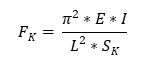Minimum piston rod diameter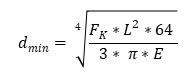Check whether Euler case applies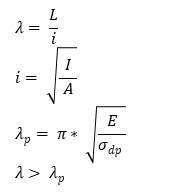F.K = Permissible piston force (N)
E = modulus of elasticity (N / mm2)
I = axial moment of inertia (mm4)
L = buckling length see below (mm)
S.K = Buckling resistance (-)
dmin = minimum piston rod diameter (mm)
λ = slenderness (-)
i = radius of gyration (mm)
A = bar cross section (mm2)
σdp = Pressure yield point (N / mm2)
F.K = Permissible piston force (N)
E = modulus of elasticity (N / mm2)
I = axial moment of inertia (mm4)
L = buckling length see below (mm)
S.K = Buckling resistance (-)
dmin = minimum piston rod diameter (mm)
λ = slenderness (-)
i = radius of gyration (mm)
A = bar cross section (mm2)
σdp = Pressure yield point (N / mm2)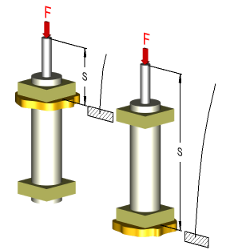Piston rod free
Cylinder clamped
L = 2 * S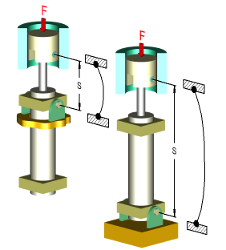Articulated piston rod
Cylinder articulated
L = S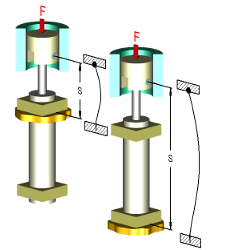Articulated piston rod
Cylinder clamped
L = S * 0.7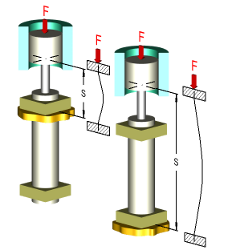Piston rod clamped
Cylinder clamped
L = S / 2

up

### Crane arm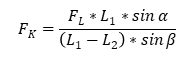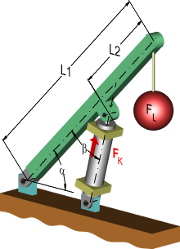F.K = Piston force (N)
L.1 = Length of the load arm (mm)
L.2 = Distance between load and cylinder (mm)
α = angle between the load arm to the horizontal (degrees)
β = angle between the load arm and the cylinder axis
F.K = Piston force (N)
L.1 = Length of the load arm (mm)
L.2 = Distance between load and cylinder (mm)
α = angle between the load arm to the horizontal (degrees)
β = angle between the load arm and the cylinder axis
up

### Lever arm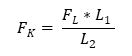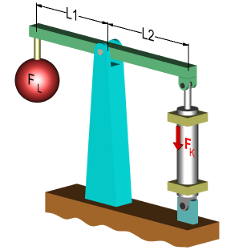F.K = Piston force (N)